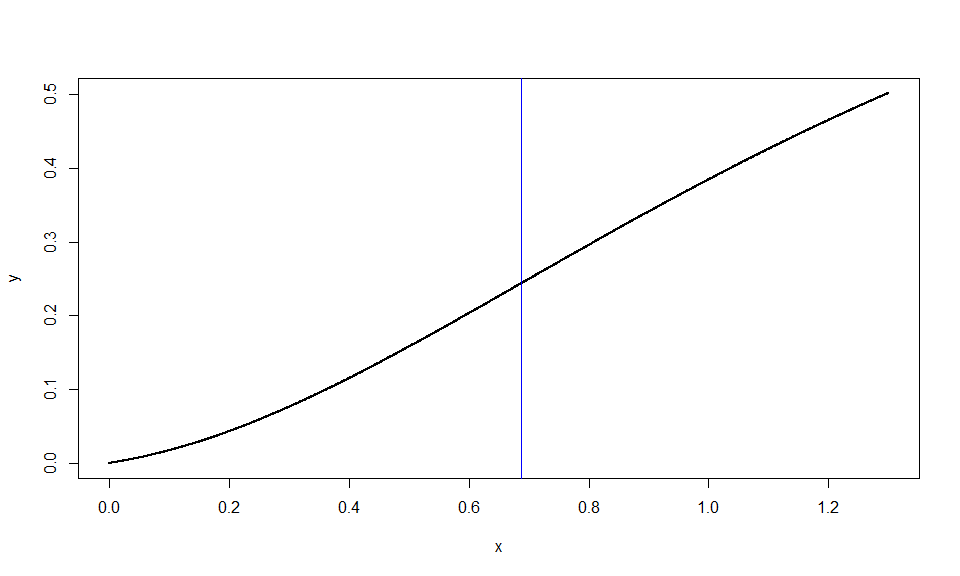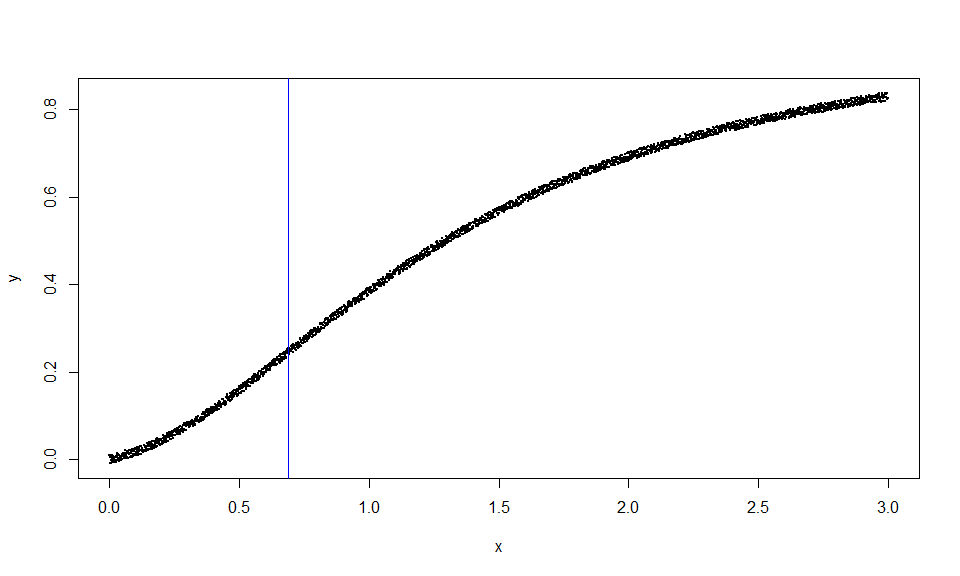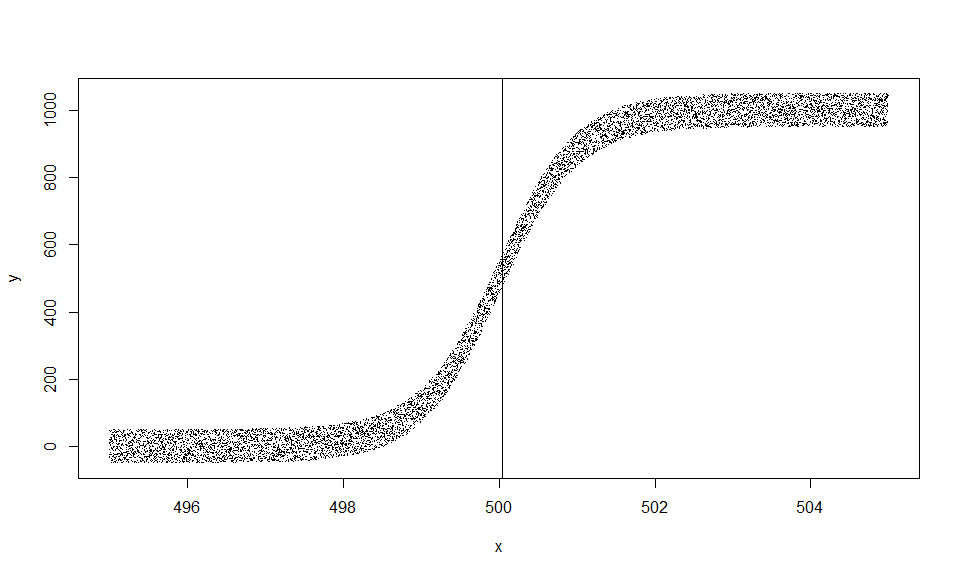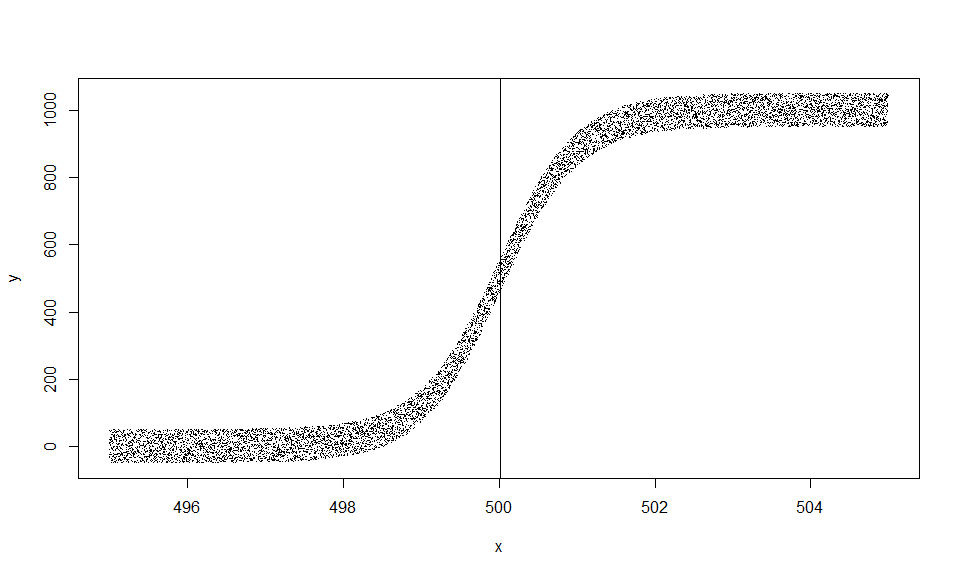# Find inflection points: Mission Impossible!

## Can we always find the inflection point?

Let’ s take the function: $f (x) = \frac{\frac{1}{8}x+\frac{1}{2}x^2}{1+\frac{1}{8}x+\frac{1}{2}x^2}$ following . What are the inflection points? We must take the first derivative: $f'(x)= \frac {8+64\,x}{ \left( 4\,{x}^{2}+x+8 \right) ^{2}}$

and continue to second derivative: $f''(x)= \frac {-768\,{x}^{2}-192\,x+496}{ \left( 4\,{x}^{2}+x+8 \right) ^{3}}$ Now we must solve above expression for x and find two solutions: $x_1=-1/8-1/24\,\sqrt {381}=-0.9383008876$ $x_2=-1/8+1/24\,\sqrt {381}=0.6883008876$ If we study the function we’ll see that has a minimum at $$x_{min}=-\frac{1}{8}=-0.125$$ and it is concave/convex at interval $$x<x_{min}$$ and convex/concave for $$x>x_{min}$$.

But what if we do not want to perform such an analytical approach? What if we just wanted the inflection points without more details?

Then we must follow next steps:

• define an interval that encloses an inflection point
• run ESE or EDE to find a first approximation
• choose an interval that encloses inflection with your desired accuracy
• run BESE to find the closest available approximation

Here are the steps for the positive inflection point:

library(inflection)
f=function(x){(1/8*x+1/2*x^2)/(1+1/8*x+1/2*x^2)}
x=seq(0,5,0.1)
y=f(x)
# First approximation
cc=check_curve(x,y);cc
## $ctype ##  "convex_concave" ## ##$index
##  0
ese(x,y,cc$index) ## j1 j2 chi ## ESE 2 13 0.65 # New interval with equal distances from first approximation x=seq(0,1.3,0.001) y=f(x) # Second approximation cc=check_curve(x,y);cc ##$ctype
##  "convex_concave"
##
## $index ##  0 ipbese=bese(x,y,cc$index)
ipbese$iplast ##  0.688 plot(x,y,pch=19,cex=0.1) abline(v=ipbese$iplast,col='blue')BESE
n a b ESE
1301 0.000 1.300 0.8090
711 0.495 0.819 0.6570
325 0.627 0.794 0.7105
168 0.638 0.720 0.6790
83 0.673 0.714 0.6935
42 0.676 0.696 0.6860
21 0.684 0.694 0.6890
11 0.685 0.690 0.6875
6 0.687 0.689 0.6880

Since our interval was defined with equal size step 0.001 we expect our estimation to have the same accuracy and indeed it is truth.

If we wanted a better accuracy, for example with 4 digits, then we could define another interval with previous approximation inside it and with equal size stpe 0.0001:

# x=seq(0.68,0.69,0.0001)
# y=f(x)
# cc=check_curve(x,y);cc
# ipbese=bese(x,y,cc$index,doparallel = TRUE) # ipbese$iplast
# #  0.6883
# ipbese$iters # n a b ESE ## 1 101 0.6800 0.6900 0.68870 ## 2 25 0.6876 0.6887 0.68815 ## 3 12 0.6881 0.6886 0.68835 ## 4 6 0.6882 0.6884 0.68830 ## What if our data set is noisy? There exist no problem. Since ESE and EDE methods both give consistent estimators for the inflection point, we do not need to worry about noise. Lets perform the same task with error added: x=seq(0.0,3.,0.001) set.seed(20190628) y=f(x)+runif(length(x),-0.01,0.01) cc=check_curve(x,y) cc ##$ctype
##  "convex_concave"
##
## $index ##  0 ipbese=bese(x,y,cc$index)
ipbese$iplast ##  0.689 plot(x,y,pch=19,cex=0.1) abline(v=ipbese$iplast,col='blue')BESE
n a b ESE
3001 0.000 3.000 0.6955
1034 0.473 0.993 0.7330
521 0.542 0.836 0.6890

If your data set gas million of cases, then EDE and BEDE are your best friends.

Lets see why by using 1000001 cases from a function with inflection point equal to 500:

f=function(x){500+500*tanh(x-500)}
x=seq(0,1000,0.001)
y=f(x)
length(x)
##  1000001
t1=Sys.time();ede(x,y,0);t2=Sys.time();t2-t1
##         j1     j2 chi
## EDE 496201 503801 500
## Time difference of 0.01299381 secs
ipbede=bede(x,y,cc$index) ipbede$iplast
##  500
BEDE
n a b EDE
1000001 0.000 1000.000 500
7601 498.712 501.288 500
2577 499.341 500.659 500
1319 499.633 500.367 500
735 499.791 500.209 500
419 499.880 500.120 500
241 499.931 500.069 500
139 499.960 500.040 500
81 499.977 500.023 500
47 499.987 500.013 500
27 499.993 500.007 500
15 499.996 500.004 500
9 499.998 500.002 500
5 499.999 500.001 500

Situation will not change if we add error $$U(-50,50)$$:

f=function(x){500+500*tanh(x-500)}
x=seq(0,1000,0.001)
set.seed(20190628)
y=f(x)+runif(length(x),-50,50)
length(x)
##  1000001
t1=Sys.time();ede(x,y,0);t2=Sys.time();t2-t1
##         j1     j2      chi
## EDE 496208 503817 500.0115
## Time difference of 0.01699495 secs
ipbede=bede(x,y,0)
ipbede$iplast ##  500.046 plot(x[495000:505000],y[495000:505000],xlab="x",ylab="y",pch='.') abline(v=ipbede$iplast)BEDE
n a b EDE
1000001 0.000 1000.000 500.0115
7610 498.815 501.277 500.0460

Even if our data are not symmetric, estimation will not change:

f=function(x){500+500*tanh(x-500)}
x=seq(0,700,0.001)
set.seed(20190628)
y=f(x)+runif(length(x),-50,50)
length(x)
##  700001
t1=Sys.time();ede(x,y,0);t2=Sys.time();t2-t1
##         j1     j2     chi
## EDE 496208 503646 499.926
## Time difference of 0.009998083 secs
ipbede=bede(x,y,0)
ipbede$iplast ##  500.013 plot(x[495000:505000],y[495000:505000],xlab="x",ylab="y",pch='.') abline(v=ipbede$iplast)BEDE
n a b EDE
700001 0.000 700.000 499.926
7439 498.829 501.197 500.013

Just try to solve above problems by using regression or other techniques that involve matrix inversion…

OK, we’ll perform that task for you.

# library(nlme)
# x=seq(0,5,0.1)
# f=function(x){(1/8*x+1/2*x^2)/(1+1/8*x+1/2*x^2)}
# y=f(x)
# df=data.frame("x"=x,"y"=y)
# Asym=1;xmid=1;scal=1;
# fmla=as.formula("y~SSlogis(x,Asym,xmid,scal)");fmla
# try(nls(fmla,df))
# est=try(nls(fmla,df))
# coef(est)

When it works it gives the result xmid=1.2516 which is away from true inflcetion (0.6883008876), altough all results seem to be statistically ‘super’:

# y ~ SSlogis(x, Asym, xmid, scal)
# Nonlinear regression model
#   model: y ~ SSlogis(x, Asym, xmid, scal)
#    data: df
#   Asym   xmid   scal
# 0.8909 1.2516 0.5478
#  residual sum-of-squares: 0.05242
#
# Number of iterations to convergence: 0
# Achieved convergence tolerance: 1.401e-06
###
# est=try(nls(fmla,df))
# coef(est)
#      Asym      xmid      scal
# 0.8908913 1.2516410 0.5478263
# summary(est)
#
# Formula: y ~ SSlogis(x, Asym, xmid, scal)
#
# Parameters:
#      Estimate Std. Error t value Pr(>|t|)
# Asym 0.890891   0.007872  113.18   <2e-16 ***
# xmid 1.251641   0.025638   48.82   <2e-16 ***
# scal 0.547826   0.023718   23.10   <2e-16 ***
# ---
# Signif. codes:  0 ‘***’ 0.001 ‘**’ 0.01 ‘*’ 0.05 ‘.’ 0.1 ‘ ’ 1
#
# Residual standard error: 0.03305 on 48 degrees of freedom
#
# Number of iterations to convergence: 0
# Achieved convergence tolerance: 1.401e-06

# x=seq(0.0,3.,0.001)
# f=function(x){(1/8*x+1/2*x^2)/(1+1/8*x+1/2*x^2)}
# set.seed(20190628)
# y=f(x)+runif(length(x),-0.01,0.01)
# df=data.frame("x"=x,"y"=y)
# Asym=1;xmid=1;scal=1;
# fmla=as.formula("y~SSlogis(x,Asym,xmid,scal)");fmla
# try(nls(fmla,df))

When it works it gives next result xmid=1.0938 which is away from true inflcetion (0.6883008876) and again rsults seem to be ‘excellent’:

# Nonlinear regression model
# model: y ~ SSlogis(x, Asym, xmid, scal)
# data: df
# Asym   xmid   scal
# 0.8018 1.0938 0.4318
# residual sum-of-squares: 1.884
#
# Number of iterations to convergence: 0
# Achieved convergence tolerance: 1.768e-07
###
# est=try(nls(fmla,df))
# coef(est)
#      Asym      xmid      scal
# 0.8018405 1.0937758 0.4318175
# summary(est)
#
# Formula: y ~ SSlogis(x, Asym, xmid, scal)
#
# Parameters:
#      Estimate Std. Error t value Pr(>|t|)
# Asym 0.801840   0.001175   682.7   <2e-16 ***
# xmid 1.093776   0.002417   452.5   <2e-16 ***
# scal 0.431817   0.002063   209.3   <2e-16 ***
# ---
# Signif. codes:  0 ‘***’ 0.001 ‘**’ 0.01 ‘*’ 0.05 ‘.’ 0.1 ‘ ’ 1
#
# Residual standard error: 0.02507 on 2998 degrees of freedom
#
# Number of iterations to convergence: 0
# Achieved convergence tolerance: 1.768e-07

We set as initial parameter (xmid) the true solution (500), but it failed to proceed:

# f=function(x){500+500*tanh(x-500)}
# x=seq(0,1000,0.001)
# y=f(x)
# length(x)
# df=data.frame("x"=x,"y"=y)
# Asym=1000;xmid=500;scal=1;
# fmla=as.formula("y~SSlogis(x,Asym,xmid,scal)");fmla
#
# t1=Sys.time();
# try(nls(fmla,df))
# Error in nls(y ~ 1/(1 + exp((xmid - x)/scal)), data = xy, start = list(xmid = aux[[1L]],  :
#   step factor 0.000488281 reduced below 'minFactor' of 0.000976562
# t2=Sys.time();t2-t1
# Time difference of 19.29784 secs

So R package inflection can give you an answer for all extremely difficult cases.